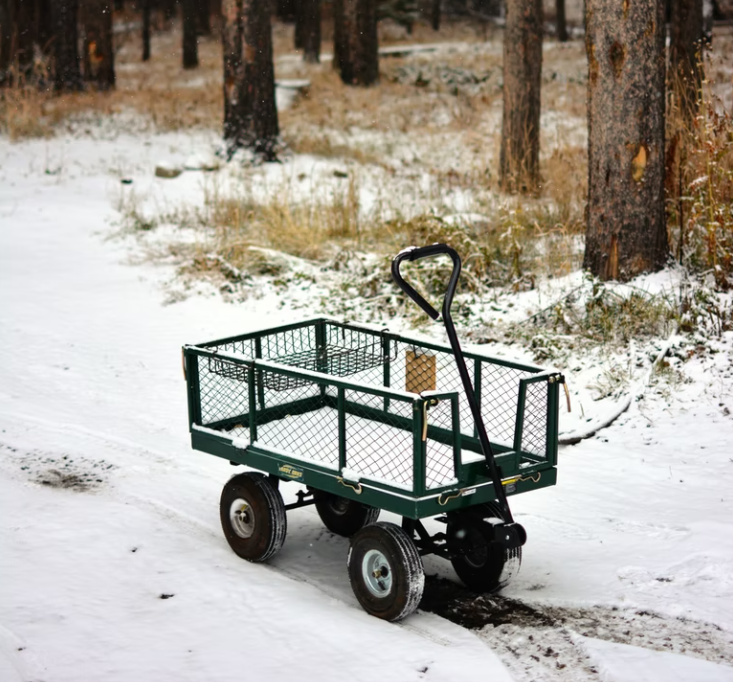## Impulse, Momentum, Accel

Consider a 0.75 kg wagon (Wagon 1) is pulled forward with a 2 N force for 2 seconds. Consider a second 0.75 kg wagon (Wagon 2) is pulled forward with a 4 N force for 1 second.
1. Does Wagon 1 or Wagon 2 have the greater acceleration?
2. Does Wagon 1 or Wagon 2 have the greater impulse?
3. Does Wagon 1 or wagon 2 have the greater momentum change?Hint
$$Force=mass\cdot acceleration=mass\cdot \frac{\Delta velocity}{\Delta time}$$$Hint 2 Impulse is defined as $$force\cdot time$$ , while momentum is defined as $$mass\cdot velocity$$ , and the change in momentum is $$mass\cdot \Delta velocity$$ . Newton's second law: $$Force=mass\cdot acceleration=mass\cdot \frac{\Delta velocity}{\Delta time}$$$
We can rearrange the above equation to solve the acceleration for both wagons:
$$a_1=\frac{F_1}{m_1}=\frac{2N}{0.75kg}=2.67\:m/s^2$$$$$a_2=\frac{F_2}{m_2}=\frac{4N}{0.75kg}=5.33\:m/s^2$$$
(1.) Wagon 2 has the greater acceleration.

Impulse is defined as $$force\cdot time$$ , while momentum is defined as $$mass\cdot velocity$$ , and the change in momentum is $$mass\cdot \Delta velocity$$ . Therefore, if we multiple both sides of the second law equation by $$\Delta t$$ :
$$Force \cdot \Delta time=mass\cdot \Delta velocity$$$$$Impulse=Change\:in\:momentum$$$
$$Impulse_1=F_1\cdot \Delta t_1=2N\cdot 2s=4\:N\cdot s$$$$$Impulse_2=F_2\cdot \Delta t_2=4N\cdot 1s=4\:N\cdot s$$$
(2.) The impulse is the same for both wagons.
(3.) Because momentum change is impulse, both wagons have the same momentum change.
(1.) Wagon 2 has the greater acceleration.
(2.) The impulse is the same for both wagons.
(3.) The momentum change is the same for both wagons.